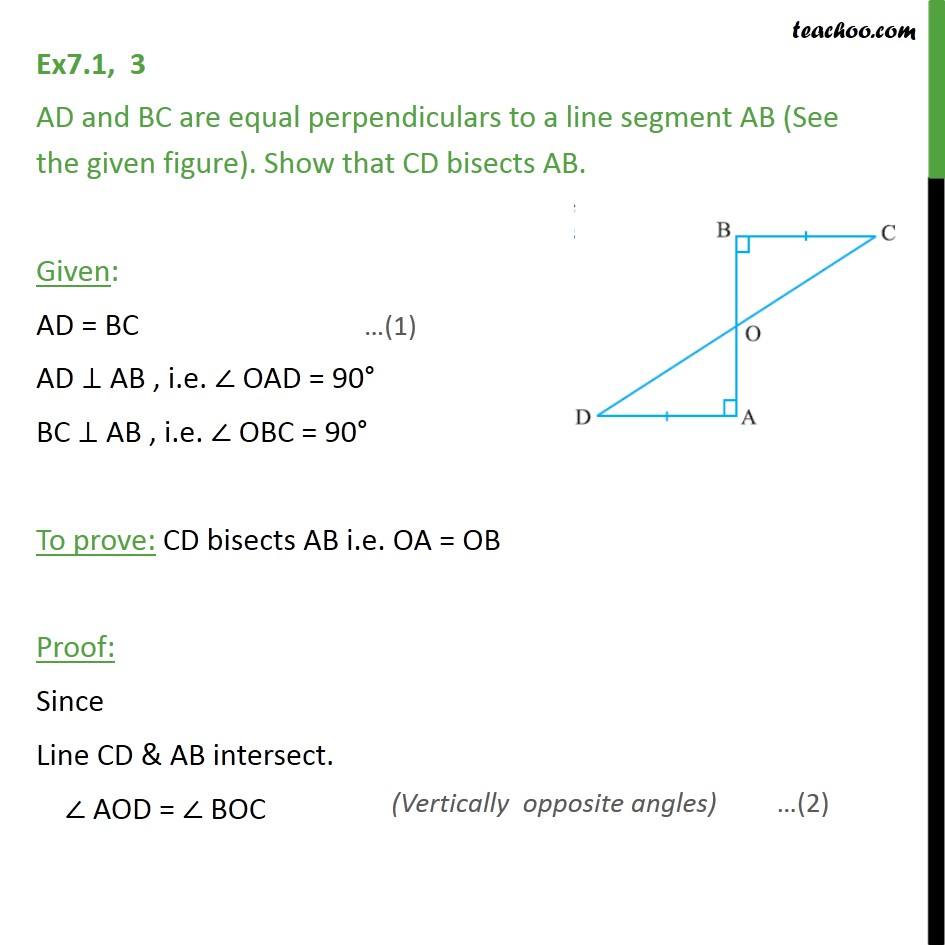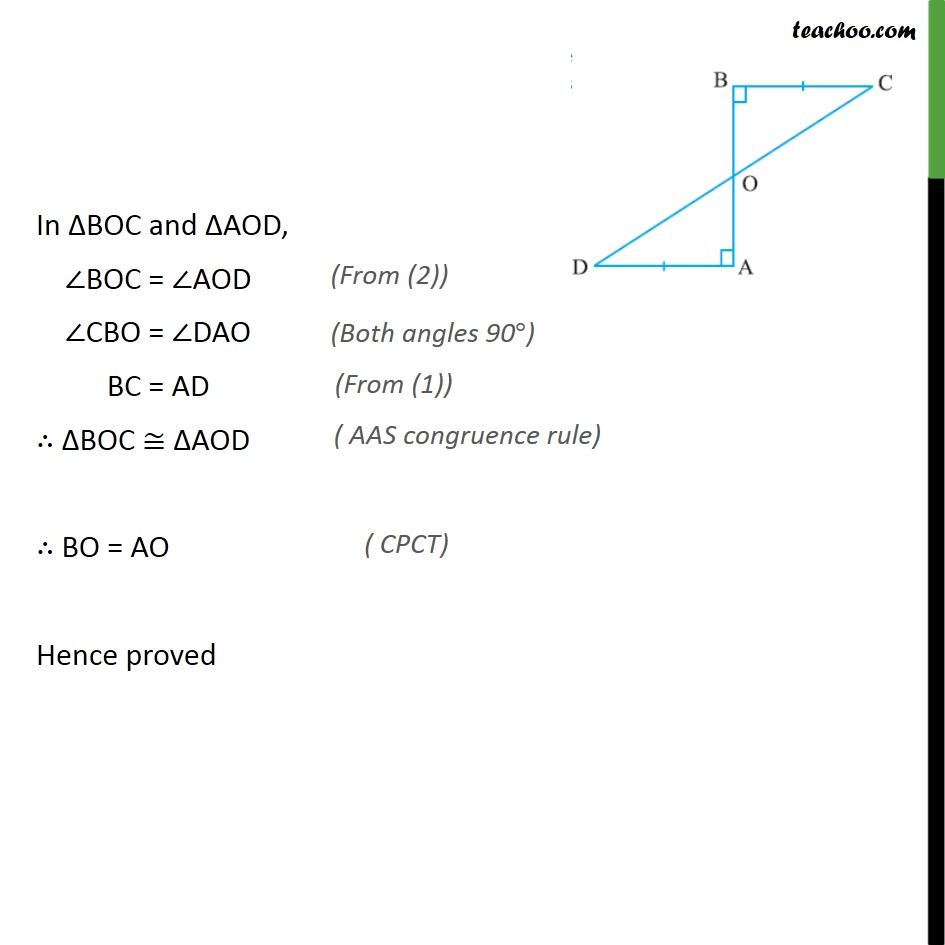1. Chapter 7 Class 9 Triangles (Term 1)
2. Concept wise
3. ASA/AAS

Transcript

Ex7.1, 3 AD and BC are equal perpendiculars to a line segment AB (See the given figure). Show that CD bisects AB. Given: AD = BC AD AB , i.e. OAD = 90 BC AB , i.e. OBC = 90 To prove: CD bisects AB i.e. OA = OB Proof: Since Line CD & AB intersect. AOD = BOC In BOC and AOD, BOC = AOD CBO = DAO BC = AD BOC AOD BO = AO Hence proved